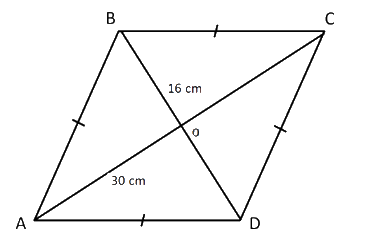# Ex.6.5 Q8 The Triangle and Its Properties - NCERT Maths Class 7

Go back to  'Ex.6.5'

## Question

The diagonals of a rhombus measure $$\rm{}16\,cm$$ and $$\rm{}30\, cm.$$ Find its perimeter.Video Solution
Triangle & Its Properties
Ex 6.5 | Question 8

## Text Solution

What is  known?

Diagonals of rhombus are given as $$\rm{}16\,cm$$ and $$\rm{}30\,cm.$$

What is unknown?

Perimeter of rhombus.

Reasoning:

This question is also based on the two concepts i.e. the concept of rhombus  and Pythagoras theorem. For better understanding of this question understand it with the help of a figure.

As it is mentioned in the question suppose there is a rhombus $$ABCD$$ and length of whose diagonals are given as $$16 \rm \,cm$$ and $$\rm{}30\,cm.$$. As, we know the diagonals of a rhombus bisect each other at $$90^\circ$$ i.e. at $$O$$. Now, $$O$$ divides $$DB$$ and $$AC$$ into two equal parts i.e. $$OD$$ is half of $$DB$$ and $$OC$$ is half $$AC.$$ Now, you can apply Pythagoras theorem in triangle $$DOC$$ and get the measure of side $$DC.$$ Now, the side of rhombus is known, you can easily find the perimeter of rhombus.

Steps

Given, $$AC$$ and $$BD$$ are the two diagonals of the rhombus.

$$AC = \rm{}30\,cm,$$ $$BD =\rm{}16\,cm$$

Since, the diagonal of a rhombus bisect each other at $$90^\circ.$$

Therefore,

\begin{align}OD &= \frac{{DB}}{2} = \frac{{16}}{2} = 8\;\rm{cm}\\OC &= \frac{{AC}}{2} = \frac{{30}}{2} = 15\;\rm{cm} \end{align}

Now, in right angled triangle$$\Delta \,DOC,$$

\begin{align}&\left( \text{Hypotenuse} \right)^2 \\&= \rm{{ }}{{\left(\text {Perpendicular} \right)}^2} + {\rm{ }}{{\left( \text{Base} \right)}^2} \end{align}

\begin{align} {{\left( {DC} \right)}^2} &= {{\left( {OD} \right)}^2} + {{\left( {OC} \right)}^2}\\{{\left( {DC} \right)}^2} &= \rm{{ }}{{\left( 8 \right)}^2} + {\rm{ }}{{\left( {15} \right)}^2}\\{{\left( {DC} \right)}^2} &= \rm{{ }}64{\rm{ }} + {\rm{ }}225\\{{\left( {DC} \right)}^2} &= {\rm{ }}289\\DC{\rm{ }} &= \rm{{ }}17{\rm{ }}\,cm\end{align}

\begin{align}\text{Perimeter of rhombus}&=\rm{}4\,\times \,{side}\\&=\rm{} 4 \,\times \,17 \\&= \rm{}68\, \rm{cm}\end{align}

Thus, the perimeter of rhombus is $$\rm{}68\,cm.$$

Useful Tip:

Whenever you encounter problem of this kind, it is best to think of the properties related to rhombus.

Learn from the best math teachers and top your exams

• Live one on one classroom and doubt clearing
• Practice worksheets in and after class for conceptual clarity
• Personalized curriculum to keep up with school